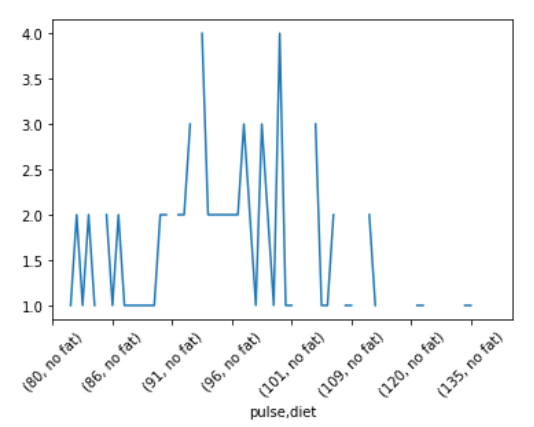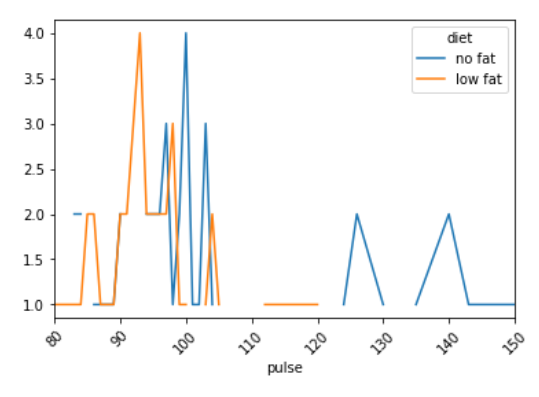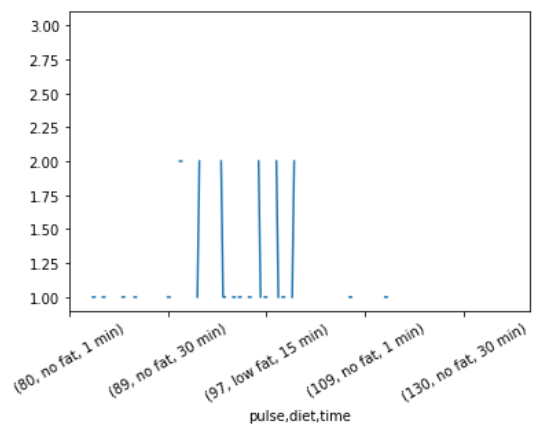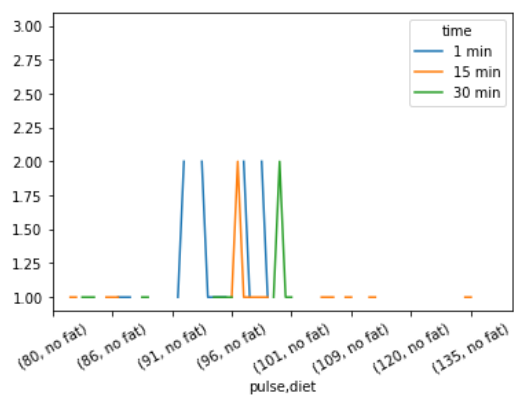GFG App
Open AppBrowser
Continue

# Pandas – Groupby multiple values and plotting results

In this article, we will learn how to groupby multiple values and plotting the results in one go. Here, we take “exercise.csv” file of a dataset from seaborn library then formed different groupby data and visualize the result.

For this procedure, the steps required are given below :

• Import libraries for data and its visualization.
• Create and import the data with multiple columns.
• Form a groupby object by grouping multiple values.
• Visualize the grouped data.

Below is the implementation with some examples :

Example 1 :

In this example, we take the “exercise.csv” file of a dataset from the seaborn library then formed groupby data by grouping two columns “pulse” and “diet” together on the basis of a column “time” and at last visualize the result.

## Python3

 `# importing packages` `import` `seaborn`   `# load dataset and view` `data ``=` `seaborn.load_dataset(``'exercise'``)` `print``(data)`   `# multiple groupby (pulse and diet both)` `df ``=` `data.groupby([``'pulse'``, ``'diet'``]).count()[``'time'``]` `print``(df)`   `# plot the result` `df.plot()` `plt.xticks(rotation``=``45``)` `plt.show()`

Output :

```    Unnamed: 0  id     diet  pulse    time     kind
0            0   1  low fat     85   1 min     rest
1            1   1  low fat     85  15 min     rest
2            2   1  low fat     88  30 min     rest
3            3   2  low fat     90   1 min     rest
4            4   2  low fat     92  15 min     rest
..         ...  ..      ...    ...     ...      ...
85          85  29   no fat    135  15 min  running
86          86  29   no fat    130  30 min  running
87          87  30   no fat     99   1 min  running
88          88  30   no fat    111  15 min  running
89          89  30   no fat    150  30 min  running

[90 rows x 6 columns]
pulse  diet
80     no fat     NaN
low fat    1.0
82     no fat     NaN
low fat    1.0
83     no fat     2.0
...
140    low fat    NaN
143    no fat     1.0
low fat    NaN
150    no fat     1.0
low fat    NaN
Name: time, Length: 78, dtype: float64```Example 2: This example is the modification of the above example for better visualization.

## Python3

 `# importing packages` `import` `seaborn`   `# load dataset` `data ``=` `seaborn.load_dataset(``'exercise'``)`   `# multiple groupby (pulse and diet both)` `df ``=` `data.groupby([``'pulse'``, ``'diet'``]).count()[``'time'``]`   `# plot the result` `df.unstack().plot()` `plt.xticks(rotation``=``45``)` `plt.show()`

Output :Example 3:

In this example, we take “exercise.csv” file of a dataset from seaborn library then formed groupby data by grouping three columns “pulse”, “diet” , and “time” together on the basis of a column “kind” and at last visualize the result.

## Python3

 `# importing packages` `import` `seaborn`   `# load dataset and view` `data ``=` `seaborn.load_dataset(``'exercise'``)` `print``(data)`   `# multiple groupby (pulse, diet and time)` `df ``=` `data.groupby([``'pulse'``, ``'diet'``, ``'time'``]).count()[``'kind'``]` `print``(df)`   `# plot the result` `df.plot()` `plt.xticks(rotation``=``30``)` `plt.show()`

Output :

```Unnamed: 0  id     diet  pulse    time     kind
0            0   1  low fat     85   1 min     rest
1            1   1  low fat     85  15 min     rest
2            2   1  low fat     88  30 min     rest
3            3   2  low fat     90   1 min     rest
4            4   2  low fat     92  15 min     rest
..         ...  ..      ...    ...     ...      ...
85          85  29   no fat    135  15 min  running
86          86  29   no fat    130  30 min  running
87          87  30   no fat     99   1 min  running
88          88  30   no fat    111  15 min  running
89          89  30   no fat    150  30 min  running

[90 rows x 6 columns]
pulse  diet     time
80     no fat   1 min     NaN
15 min    NaN
30 min    NaN
low fat  1 min     1.0
15 min    NaN
...
150    no fat   15 min    NaN
30 min    1.0
low fat  1 min     NaN
15 min    NaN
30 min    NaN
Name: kind, Length: 234, dtype: float64```Example 4: This example is the modification of the above example for better visualization.

## Python3

 `# importing packages` `import` `seaborn`   `# load dataset` `data ``=` `seaborn.load_dataset(``'exercise'``)`   `# multiple groupby (pulse, diet, and time)` `df ``=` `data.groupby([``'pulse'``, ``'diet'``, ``'time'``]).count()[``'kind'``]`   `# plot the result` `df.unsatck().plot()` `plt.xticks(rotation``=``30``)` `plt.show()`

Output :My Personal Notes arrow_drop_up